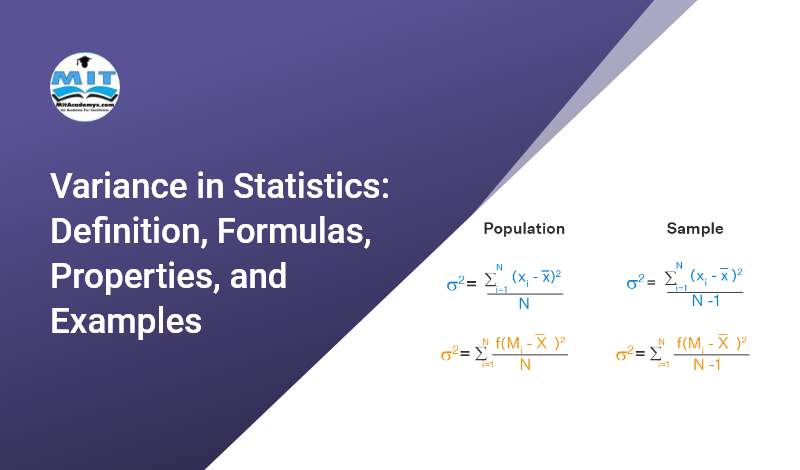## Variance in Statistics: Definition, Formulas, Properties, and Examples

The variance is a widely used concept in probability and descriptive statistics for measuring the variability of the given data. The variance is a technique for determining the various terms in finance. It is just the same as the standard deviation but it is measured in squared units. Let us explore the term variance. What …